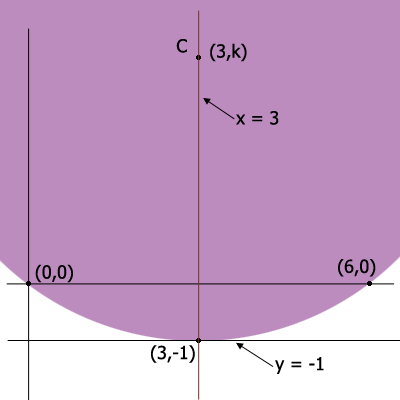SEARCH HOMEMath Central Quandaries & QueriesQuestion from shahad, a student: question 1 find an equation for the circle through the point (0,0) and (6,0) that a tangent to the line y=-1 question 2 find an equation for the circle through the point (0,0) and (17,7) whose center lies on the line 12x-5y=0I'll give you some help with problem 1, a similar approach works with problem 2.

In problem 1 the center $C$ of the circle must be on the line $x = 3.$ Thus $C$ has coordinates $(3, k)$ for some number $k.$Thus the distance from $C$ to $(0, 0)$ must equal the distance from $C$ to $(3, -1).$

Solve for $k.$

PennyMath Central is supported by the University of Regina and The Pacific Institute for the Mathematical Sciences.